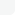# CategoriesNitroflare
Rapidgator

Become VIP Users

# Mobile / For Android →Graphing Calculator - Algeo v2.31Requirements: Android 5.0+ | File size: 13,82 MB

Use Algeo as a physics calculator, a scientific calculator, an algebra calculator, and a chemistry calculator! Algeo is the most beautiful scientific graphing calculator available on the Play Store. It's fast and powerful and you'll never have to carry around a large physical scientific calculator anymore. The intuitive interface shows your graphing calculation as you would write it on paper rather than squeezing everything on a single line. And you don't need an internet connection unlike other graphing calculators! Useful for calculus, physics or as a chemistry calculator.
Wolfram Alpha users love using Algeo! This free app is packed with more features than a paid physics calculator. Graph fast with Algeo.
Draw functions, find intersections and show a table of values of the functions with an easy to use interface.
Use Algeo as a
• Graphing Calculator
• Physics Calculator
• Scientific Calculator
• Algebra Calculator
• Calculus Calculator
• Chemistry Calculator
As a calculus calculator
• Symbolic Differentiation
• Calculate Integrals (definite only)
• Calculate Taylor-series
• Solve Equations
• Algeo is the most advanced calculus calculator on the Google Play Store!
As a scientific calculator
• Trigonometric and Hyperbolic Functions
• Logarithm
• Result History
• Variables
• Scientific Notation
• Combinatorial functions
• Number theoretic functions (modulo, greatest common divisor)
• Use Algeo as your go-to algebra calculator!
As a graphing calculator
• Graph fast!
• Graph up to four functions
• Analyze function
• Find roots and intersections automatically
• Pinch to zoom
• Create a table of values for a function

[related-news]

## Related News

{related-news}
[/related-news]